﻿ 基于回声状态网络的船舶摇荡连续预报方法研究
 舰船科学技术2016, Vol. 38Issue (6): 67-69PDF

1. 大连海事大学 航海学院, 辽宁 大连 116026 ;
2. 大连舰艇学院, 辽宁 大连 116018

Continuous forecasting of ship sway based on ESNs
SHI Bo-wen1, ZHANG Xin-yu2, LIU Zheng-jiang1
1. Navigation College, Dalian Maritime University, Dalian 116026, China ;
2. Dalian Naval Academy, Dalian 116018, China
Abstract: Echo state networks (ESNs) is a new kind of recurrent neural networks, which is suitable for the data processing based on finite number of training samples to forecast the unknown data by a nolinear model. It has preponderance for solving the nonlinearity problems. Without prior information of sea waves and the state equations of ship motions, only using the real measured roll and pitch data, the ESNs method is applied to solve the problem of short time series forecasting. Results show that the method satisfies the need of online forecasting within 15 seconds, and continuous forecasting can be realized by sliding the window.
Key words: echo state networks     ship sway     continuous forecasting
0 引言

1 船舶摇荡数据的采集与分析 1.1 实验设备介绍

1.2 实验概况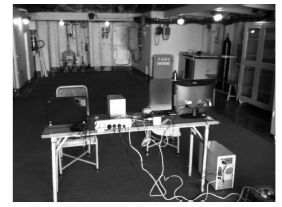图 1 测姿实验现场 Fig. 1 Motion measurement equipment
2 回声状态网络

 $u(n) = {[{u_1}(n),{u_2}(n),\cdots ,{u_p}(n)]^{\rm T}}\text{，}$

i 个输出单元：

 $y(n) = {[{y_1}(n),{y_2}(n),\cdots ,{y_i}(n)]^{\rm T}}\text{，}$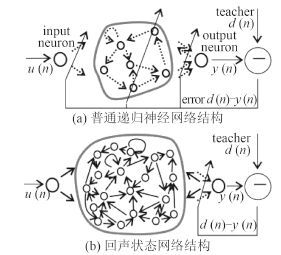图 2 回声状态网络与普通递归神经网络对比 Fig. 2 Comparison of Echo state networks and normal recurrent neural networks

 $x(n + 1) \!=\! f(W_{res}^{in}u(n \!+\! 1) \!+\! {W_{res}}x(n) \!+\! W_{res}^{back}y(n))\text{，}$ (1)

 $y(n) = {W^{out}}x(n)\text{。}$ (2)

3 船舶摇荡的连续预报方法

 $\bar x = \frac{{{x_i}-{x_{\min }}}}{{{x_{\max }}-{x_{\min }}}}\text{，}$ (3)

 ${x_i} = \left( {{x_{\max }}-{x_{\min }}} \right)\bar x + {x_{\min }}\text{。}$ (4)

4.3 预报效果分析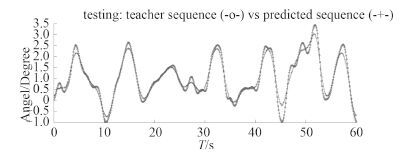图 3 对某船横摇数据连续预报结果 Fig. 3 Continuous roll forecasting of a target ship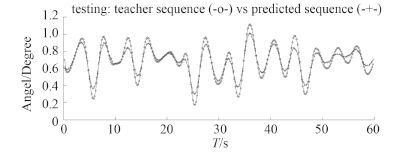图 4 某训练船纵摇数据连续预报结果 Fig. 4 Continuous pitch forecasting of a training ship
4.4 评价指标

 $SMAPE = \frac{{\left| {{x_{m + i}}-x_{m + i}^ * } \right|}}{{{x_{1/3}}}}\text{。}$ (5)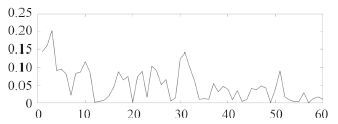图 5 某船横摇数据预报连续 15 秒时，相对三一横摇值的误差 Fig. 5 The relative error corresponding to significant roll data of a target ship while performing 15 seconds forecasting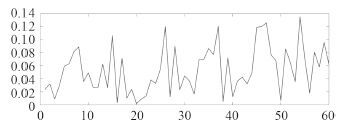图 6 某训练船纵摇数据连续 15 秒时，相对三一纵摇值的误差 Fig. 6 The relative error corresponding to significant pitch data of a training ship while performing 15 seconds forecasting
5 结语

  刘孙波, 施平安, 陈聚和. 舰船波浪中运动时域预报方法研究[J]. 广州航海学院学报 , 2014, 22 (2) :21–23.  彭秀艳, 赵希人, 魏纳新, 等. 大型舰船姿态运动极短期预报的一种AR算法[J]. 船舶工程 , 2001 (5) :5–7.  彭秀艳, 刘长德. 基于格型递归最小二乘算法的船舶运动极短期预报[J]. 船舶力学 , 2012, 16 (1/2) :44–51.  王琳. 基于CMAC神经网络的舰船运动预报仿真研究[J]. 数学的实践与认识 , 2014, 44 (23) :160–165.  刘博, 蔡烽, 石爱国. 舰船摇荡预报中的双变量局域线性多步预报法[J]. 中国航海 , 2012, 35 (2) :33–38.  陈远明, 叶家玮, 张兮龙. 船舶运动的极短期预报试验[J]. 船海工程 , 2010, 39 (1) :13–15.  侯建军, 常小勇, 石爱国. 舰船摇荡运动数据采集及混沌特性分析[J]. 船舶工程 , 2011, 33 (6) :25–28.  JAEGER H, HAAS H. Harnessing nonlinearity:predicting chaotic systems and saving energy in wireless communication[J]. Science , 2004, 304 (5667) :78–80. DOI:10.1126/science.1091277  郭嘉. 回声状态网络分类方法及其应用研究[D]. 哈尔滨:哈尔滨工业大学, 2011.